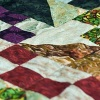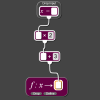# Resources tagged with: Factors and multiples

Filter by: Content type:
Age range:
Challenge level:

### There are 154 results

Broad Topics > Properties of Numbers > Factors and multiples### X Marks the Spot

##### Age 11 to 14 Challenge Level:

When the number x 1 x x x is multiplied by 417 this gives the answer 9 x x x 0 5 7. Find the missing digits, each of which is represented by an "x" .##### Age 11 to 14 Challenge Level:

Make a set of numbers that use all the digits from 1 to 9, once and once only. Add them up. The result is divisible by 9. Add each of the digits in the new number. What is their sum? Now try some. . . .### Just Repeat

##### Age 11 to 14 Challenge Level:

Think of any three-digit number. Repeat the digits. The 6-digit number that you end up with is divisible by 91. Is this a coincidence?### Big Powers

##### Age 11 to 16 Challenge Level:

Three people chose this as a favourite problem. It is the sort of problem that needs thinking time - but once the connection is made it gives access to many similar ideas.### Repeaters

##### Age 11 to 14 Challenge Level:

Choose any 3 digits and make a 6 digit number by repeating the 3 digits in the same order (e.g. 594594). Explain why whatever digits you choose the number will always be divisible by 7, 11 and 13.### Funny Factorisation

##### Age 11 to 16 Challenge Level:

Using the digits 1 to 9, the number 4396 can be written as the product of two numbers. Can you find the factors?### Gabriel's Problem

##### Age 11 to 14 Challenge Level:

Gabriel multiplied together some numbers and then erased them. Can you figure out where each number was?### Times Right

##### Age 11 to 16 Challenge Level:

Using the digits 1, 2, 3, 4, 5, 6, 7 and 8, mulitply a two two digit numbers are multiplied to give a four digit number, so that the expression is correct. How many different solutions can you find?##### Age 11 to 16 Challenge Level:

The items in the shopping basket add and multiply to give the same amount. What could their prices be?### A First Product Sudoku

##### Age 11 to 14 Challenge Level:

Given the products of adjacent cells, can you complete this Sudoku?### Can You Find a Perfect Number?

##### Age 7 to 14### Counting Factors

##### Age 11 to 14 Challenge Level:

Is there an efficient way to work out how many factors a large number has?### Three Times Seven

##### Age 11 to 14 Challenge Level:

A three digit number abc is always divisible by 7 when 2a+3b+c is divisible by 7. Why?### American Billions

##### Age 11 to 14 Challenge Level:

Play the divisibility game to create numbers in which the first two digits make a number divisible by 2, the first three digits make a number divisible by 3...### Even So

##### Age 11 to 14 Challenge Level:

Find some triples of whole numbers a, b and c such that a^2 + b^2 + c^2 is a multiple of 4. Is it necessarily the case that a, b and c must all be even? If so, can you explain why?### Eminit

##### Age 11 to 14 Challenge Level:

The number 8888...88M9999...99 is divisible by 7 and it starts with the digit 8 repeated 50 times and ends with the digit 9 repeated 50 times. What is the value of the digit M?### Thirty Six Exactly

##### Age 11 to 14 Challenge Level:

The number 12 = 2^2 � 3 has 6 factors. What is the smallest natural number with exactly 36 factors?### Inclusion Exclusion

##### Age 11 to 14 Challenge Level:

How many integers between 1 and 1200 are NOT multiples of any of the numbers 2, 3 or 5?### What Do You Need?

##### Age 7 to 11 Challenge Level:

Four of these clues are needed to find the chosen number on this grid and four are true but do nothing to help in finding the number. Can you sort out the clues and find the number?### Factoring Factorials

##### Age 11 to 14 Challenge Level:

Find the highest power of 11 that will divide into 1000! exactly.### Divisibility Tests

##### Age 11 to 16### The Remainders Game

##### Age 7 to 14 Challenge Level:

Play this game and see if you can figure out the computer's chosen number.### Alison's Quilt

##### Age 11 to 14 Challenge Level:

Nine squares are fitted together to form a rectangle. Can you find its dimensions?### Surprising Split

##### Age 7 to 11 Challenge Level:

Does this 'trick' for calculating multiples of 11 always work? Why or why not?### Counting Cogs

##### Age 7 to 14 Challenge Level:

Which pairs of cogs let the coloured tooth touch every tooth on the other cog? Which pairs do not let this happen? Why?### Becky's Number Plumber

##### Age 7 to 11 Challenge Level:

Becky created a number plumber which multiplies by 5 and subtracts 4. What do you notice about the numbers that it produces? Can you explain your findings?### Exploring Simple Mappings

##### Age 11 to 14 Challenge Level:

Explore the relationship between simple linear functions and their graphs.### Missing Multipliers

##### Age 7 to 14 Challenge Level:

What is the smallest number of answers you need to reveal in order to work out the missing headers?### Factor Track

##### Age 7 to 14 Challenge Level:

Factor track is not a race but a game of skill. The idea is to go round the track in as few moves as possible, keeping to the rules.### Transposition Cipher

##### Age 11 to 16 Challenge Level:

Can you work out what size grid you need to read our secret message?### What Numbers Can We Make Now?

##### Age 11 to 14 Challenge Level:

Imagine we have four bags containing numbers from a sequence. What numbers can we make now?### Number Families

##### Age 11 to 14 Challenge Level:

How many different number families can you find?### Multiples Sudoku

##### Age 11 to 14 Challenge Level:

Each clue in this Sudoku is the product of the two numbers in adjacent cells.### Satisfying Statements

##### Age 11 to 14 Challenge Level:

Can you find any two-digit numbers that satisfy all of these statements?### Product Sudoku

##### Age 11 to 16 Challenge Level:

The clues for this Sudoku are the product of the numbers in adjacent squares.### Hot Pursuit

##### Age 11 to 14 Challenge Level:

I added together the first 'n' positive integers and found that my answer was a 3 digit number in which all the digits were the same...### Powerful Factorial

##### Age 11 to 14 Challenge Level:

6! = 6 x 5 x 4 x 3 x 2 x 1. The highest power of 2 that divides exactly into 6! is 4 since (6!) / (2^4 ) = 45. What is the highest power of two that divides exactly into 100!?### Oh! Hidden Inside?

##### Age 11 to 14 Challenge Level:

Find the number which has 8 divisors, such that the product of the divisors is 331776.### Remainder

##### Age 11 to 14 Challenge Level:

What is the remainder when 2^2002 is divided by 7? What happens with different powers of 2?##### Age 11 to 14 Challenge Level:

List any 3 numbers. It is always possible to find a subset of adjacent numbers that add up to a multiple of 3. Can you explain why and prove it?### Remainders

##### Age 7 to 14 Challenge Level:

I'm thinking of a number. My number is both a multiple of 5 and a multiple of 6. What could my number be?### Dozens

##### Age 7 to 14 Challenge Level:

Do you know a quick way to check if a number is a multiple of two? How about three, four or six?### Two Much

##### Age 11 to 14 Challenge Level:

Explain why the arithmetic sequence 1, 14, 27, 40, ... contains many terms of the form 222...2 where only the digit 2 appears.### Being Resilient - Primary Number

##### Age 5 to 11 Challenge Level:

Number problems at primary level that may require resilience.### Special Sums and Products

##### Age 11 to 14 Challenge Level:

Find some examples of pairs of numbers such that their sum is a factor of their product. eg. 4 + 12 = 16 and 4 × 12 = 48 and 16 is a factor of 48.### Crossings

##### Age 7 to 11 Challenge Level:

In this problem we are looking at sets of parallel sticks that cross each other. What is the least number of crossings you can make? And the greatest?### Multiplication Series: Number Arrays

##### Age 5 to 11

This article for teachers describes how number arrays can be a useful representation for many number concepts.### Money Measure

##### Age 7 to 11 Challenge Level:

How can you use just one weighing to find out which box contains the lighter ten coins out of the ten boxes?### Star Product Sudoku

##### Age 11 to 16 Challenge Level:

The puzzle can be solved by finding the values of the unknown digits (all indicated by asterisks) in the squares of the $9\times9$ grid.### Flow Chart

##### Age 11 to 14 Challenge Level:

The flow chart requires two numbers, M and N. Select several values for M and try to establish what the flow chart does.